Home | | Maths 11th std | Derivatives of basic elementary functions - Differentiation Rules

# Derivatives of basic elementary functions - Differentiation Rules

We shall now find the derivatives of all the basic elementary functions; we start with the constant function.

Derivatives of basic elementary functions

We shall now find the derivatives of all the basic elementary functions; we start with the constant function.

(1) The derivative of a constant function is zero.

Let y = f(x) = k, k is a constant.

Then f(x +∆x) = k and

f(x + x) - f(x) = k - k = 0.(2) The power function y = xn, n > 0 is an integer.

Let f(x) = xn.

Then, f(x+ ∆x) = (x + ∆x)n and

f(x + x) - f(x) = (x + x)n - xn.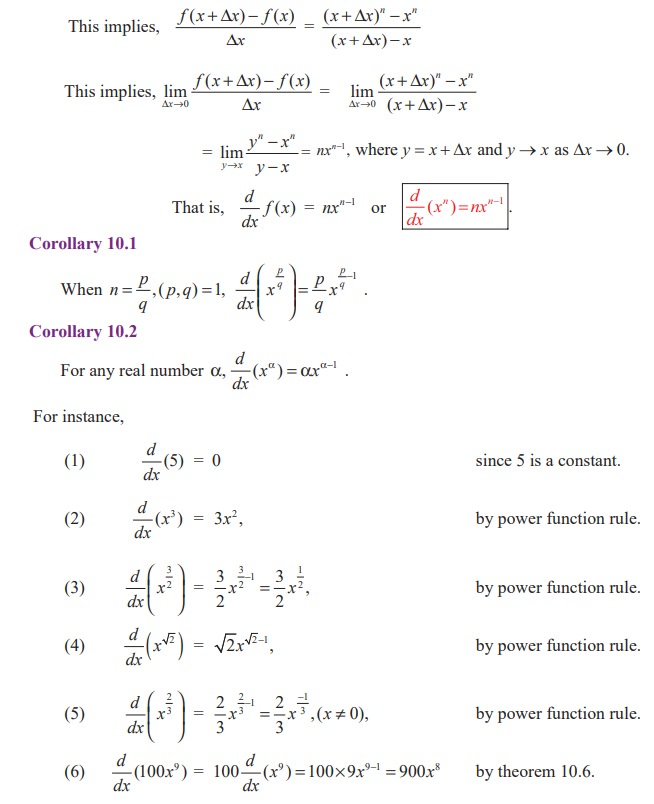(3) Derivative of the logarithmic function

The natural logarithm of x is denoted by loge x or logx or ln x

Let y =  f (x) = log x

Now  f (x + ∆x) = log (x + ∆x) and

f(x + x) - f(x) = log (x + ∆x) - log x(4) Derivative of the exponential function

Let y = ax

Then f (x + x) - f (x) = ax xax

= ax (a x−1) and(5) The derivatives of the Trigonometric functions

(i) The sine function, sinx.

Let y = f (x ) = sin x .

Then f (x + x) = sin(x + x) and(ii) The cosine function, cos x(iii) The tangent function, tan x

Let y = f (x) = tan x .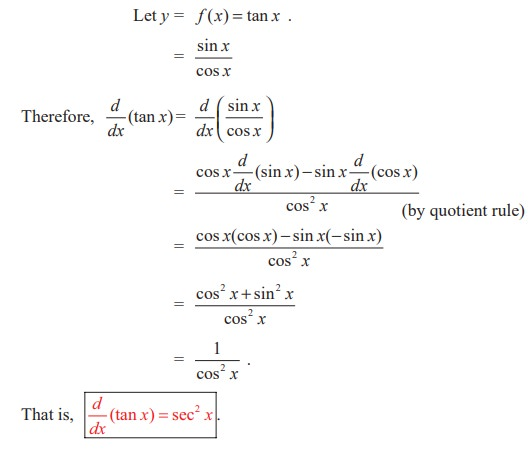(iv) The secant function,  sec x(v) The cosecant function, cosec x(vi) The cotangent function, cot x(6) The derivatives of the inverse trigonometric functions

(i) The derivative of arcsin x or sin−1 x

Let y= f (x) = sin−1 x .

Then  y + y= f (x + x ) = sin−1 (x +  x)

This implies, x= sin y and

x + x= sin (y + ∆y).(ii) The derivative of arccos x or cos1x

We know the identity :(iii) The derivative of arctan x or tan−1 x

Let y = f (x ) = tan−1 x .            ... (1)

This implies, y + y= f(x + ∆x) = tan−1 (x + ∆x)          ... (2)

x= tan y and

x+ ∆x = tan (y + ∆y)

This implies, ∆x = tan (y + ∆y) - tan y(iv) The derivative of arccot x or cot1 x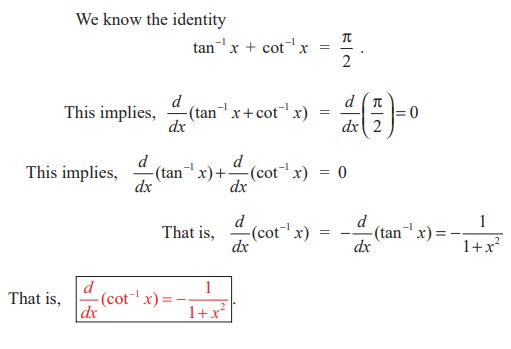(v) The derivative of arcsec x or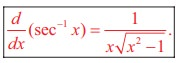(vi) The derivative of arccosec x orThe proofs of (v) and (vi) are left as exercises.EXERCISE 10.2

Find the derivatives of the following functions with respect to corresponding independent variables:

(1) f(x) = x - 3 sinx

(2) y = sin x + cos x

(3) f(x) = x sin x

(4) y = cos x - 2 tan x

(5) g(t) = t3cos t

(6) g(t) = 4 sec t + tan t

(7) y = ex sin x

(8) y = tanx /x

(9) y = sin x / [1+ cos x]

(10) y = x / [sin x + cos x]

(11) y = tan x −1 / sec x

(12) y = sin x / x2

(13) y = tan θ(sin θ + cos θ)

(14) y = cosec x . cot x

(15) y = x sin x cos x

(16) y = e-x. log x

(17) y = (x2 +5) log(1+x)e-3x

(18) y = sin x°

(19) y = log10  x

(20) Draw the function f '(x) if f (x) = 2x2 − 5x + 3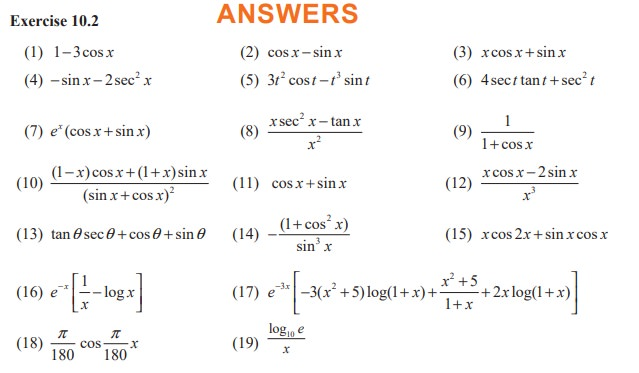Study Material, Lecturing Notes, Assignment, Reference, Wiki description explanation, brief detail
11th Mathematics : UNIT 10 : Differential Calculus: Differentiability and Methods of Differentiation : Derivatives of basic elementary functions - Differentiation Rules |